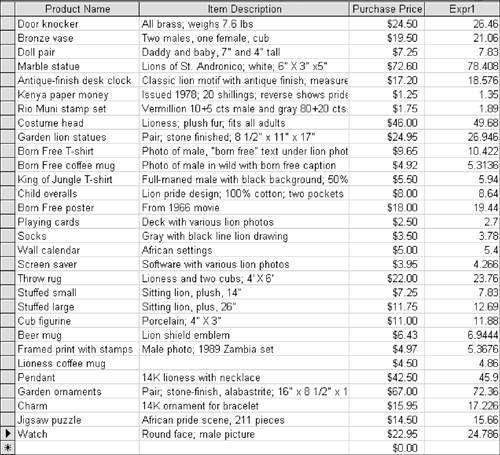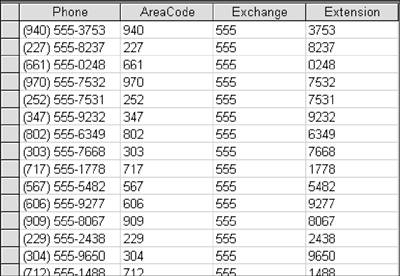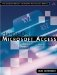# Adding Calculated Fields

Queries give you the freedom to both retrieve records and manipulate them. Notably, you can add fields that display the results of a calculation on stored values.

#### Using an Arithmetic Expression

For example, suppose you were anticipating that purchase prices would rise uniformly for all products about 8%. You want to create a field that will show what the new prices will be for each product.

You've used expressions to set criteria; now you'll use one to create a calculated field.

1.

Select the tblMerchandise table in the Database window. Choose Insert, Query. With Design View selected, click OK.

2.

Add MerchName, MerchDescription, and PurchasePrice to the design grid.

3.

Click in the Field row of the fourth column.

4.

Enter an expression as follows:

a. Type [PurchasePrice]. You enclose the field name in brackets.

b. Type *. An asterisk is the operator for multiplication.

c. Type 1.08. You want 100% of the original price plus an 8% increment. 108% expressed as a number is 1.08.

The complete expression is [PurchasePrice]*1.08.

5.

Click View to go to Datasheet view (see Figure 8.3). The numbers seem reasonable, but they are not expressed as currency. Also, the column name of Expr1 is unhelpful.

##### Figure 8.3. The field with recalculated prices has been added, but it needs to be formatted. It lacks an informative caption, and the values should be expressed as currency.6.

Click View to return to Design view. Click in the Field row of the Expr1 column. Press Shift+F2 to open the Zoom window. Edit Expr1: to ExpectedPrice. Click OK to close the Zoom window.

7.

Right-click the column and choose Properties.

Because this is a new field, there are no properties from an underlying table to inherit, so you need to set properties on your own.

8.

Click in Format, open the list, and choose Currency.

9.

Click in Caption. Type Expected Price. Close the property sheet.

10.

Click View. The column heading now shows the caption Expected Price and the figures are expressed as currency.

11.

Choose File, Save. Save the query as qryExpectedPrices. Close the query.

NOTE

Formatting the column as currency does not change its values, which sometimes extend beyond two decimal places (see Figure 8.3). In recent versions of Access, you can use the Round function to round to the intended number of decimal places. The Round function is discussed in the Microsoft Knowledge Base article 210564.

#### Using Expressions with Functions

If you looked at the glossary of expression terms in Chapter 7, you might recall that a function returns a value based on the results of a calculation or some other expression. Aggregate functions are particularly useful for findings sums, averages, and other calculations.

I discuss aggregate functions in Chapter 9, but let's first take a look at a few other functions to see how they work. One useful set of functions is Left, Mid, and Right, which let you extract parts of text values. These can be helpful in extricating, say, the most meaningful part of an account or parts number from a long text string.

Let's use the Phone field in the Customers table as an example. Let's say you want to create three columns that will extract the three-number area code, the three-number exchange, and the four-number extension.

1.

Select the tblCustomers table in the Database window. Choose Insert, Query and, with Design view selected, click OK. Maximize the window.

2.

Add CustPhone to the grid.

3.

In the first row of the second column, type AreaCode: Left ([CustPhone], 3), where:

• AreaCode is the column name.

• Left is the function that tells Access to extract values, beginning from the first character on the left.

• [CustPhone] is the field.

• 3 is the number of characters to extract.

4.

In the first row of the third column, type Exchange: Mid([CustPhone],4, 3), where:

• Exchange is the column name.

• Mid is the function.

• [CustPhone] is the field.

• 4 is the position of the first character as counted from the left.

• 3 is the number of characters to extract.

5.

In the first row of the fourth column, type Extension: Right ([CustPhone], 4).

The Right function works the same as the Left function, except that you start extracting characters from the right. There are four numbers in the extension, compared with three in an area codehence the different numbers in the expressions.

6.

Choose File, Save; name the query qryExtractFunctions and click OK.

7.

Click View to see your records (see Figure 8.4). Close the query.

##### Figure 8.4. A sample of the records extracted from the Left, Mid, and Right functions.## Q&A

 Q1: Wait a minute. If the Left function is supposed to fetch the first three characters starting from the left, why are there three numbers in the Area Code column? Isn't the first character a beginning parenthesis? Wouldn't that make the first column have strings such as (94, (22, and (66? A1: Only the nine digits of the phone number are stored in the underlying table; the parentheses and dashes are not. If these symbols had been stored as well, you'd be exactly right: The expression Left ([CustPhone], 3) would have yielded in the first row of the second column (94 instead of 940. In this case, you would want to use the Mid function to extract the area code. The expression would be Mid([CustTelephone],2,3) because 2 is the position of the first character you want as counted from the left (namely, the first number of the area code) and 3 is the number of characters you want to grab.

## Back in Algebra Class

Suppose Acme Widget determines its unit selling prices by adding \$2 to its unit purchase price and multiplying the amount by 140%, or 1.4. You're Acme's chief accountant. How do you write this expression so that Access understands it?

One reasonable possibility would seem to be [PurchasePrice]+2*1.4. But assume that the purchase price is \$3. The expression might evaluate to \$7: 3+2=5 and then 5*1.4=7. But it might also evaluate to \$5.80: 3+(1.4*2) =5.8.

Here are two rules that will help you write calculated expressions:

• Access performs any operation within parentheses first.

• Access performs multiplication and division before addition and subtraction.

According to the first rule, if you write the expression as ([PurchasePrice]+2)*1.4, Access adds the \$2 to the purchase price first and then multiplies it by 1.4. This is the expression you want.

According to the second rule, if you write the expression as [PurchasePrice]+2*1.4, Access multiplies 2 by 1.4 first and then adds it to the purchase price. This is the expression you don't want.

Suppose that, instead, you want to take 140% of the purchase price first and then add \$2 to it. You could write the expression as [PurchasePrice]*1.4+2. Access does the multiplication first and adds the \$2 afterward. But to be on the safe side and to make things clearer, your best solution is to include parentheses and enter ([PurchasePrice]*1.4)+2.Hands-On Microsoft Access: A Practical Guide to Improving Your Access Skills
ISBN: 0321245458
EAN: 2147483647
Year: 2005
Pages: 169
Authors: Bob Schneider

flylib.com © 2008-2017.
If you may any questions please contact us: flylib@qtcs.net